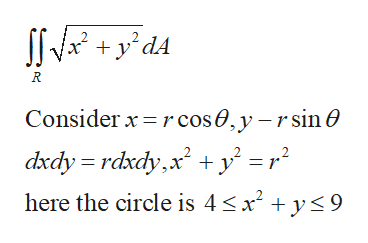# Find ʃR sqrt((x2 + y2)) dA where R is the region 4 ≤ x2 + y2 ≤ 9. Hint: Use polar coordinates.

Question
14 views

Find ʃR sqrt((x2 + y2)) dA where R is the region 4 ≤ x2 + y2 ≤ 9. Hint: Use polar coordinates.

check_circle

Step 1

Given double int...help_outlineImage TranscriptioncloseSS* +y°d4 Consider x =rcos0,y -r sin O dxdy = rdxdy,x +y' =r° here the circle is 4

### Want to see the full answer?

See Solution

#### Want to see this answer and more?

Solutions are written by subject experts who are available 24/7. Questions are typically answered within 1 hour.*

See Solution
*Response times may vary by subject and question.
Tagged in

### Calculus